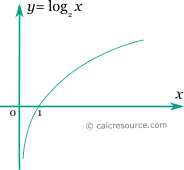## Binary logarithm calculator

This tool evaluates the binary logarithm of a number: . Enter the argument x below.

 x = Result: \log_{2} {x} =## Definitions

### General

The binary logarithm of a number x, is a power p so that 2p= x. In modern notation binary logarithm is written as . Any positive real number has a binary logarithm. The binary logarithm of 1 is 0, while the binary logarithm of 0 approaches negative infinity.

The graph of the binary logarithm function is shown in the figure below. It is a monotonic function.### Properties

The derivative of the binary logarithm function is:

The integral of the binary logarithm function is given by: